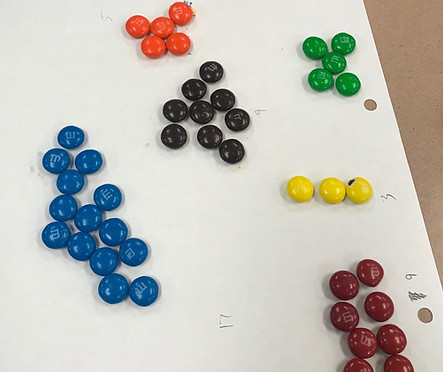## Day 113 - Lesson 11.1

##### Learning Targets
• State appropriate hypotheses and compute the expected counts and chi-square test statistic for a chi-square test for goodness of fit.

• Calculate the degrees of freedom and P-value for a chi-square test for goodness of fit.

##### Activity:

We start this lesson by telling students that we emailed the company that makes M&Ms asking about the color distribution. The company replied, claiming the following distribution:

Brown 13%, Yellow 14%, Orange 20%, Green 16%, Blue 24%, and Red 13%.

We are going to take a sample to try and find evidence against this claim. We buy one large bag of M&Ms and tell students to think of this bag as being a random sample of the entire population of M&Ms. We give each student a small handful of candies until the bag is empty, then we collect totals on the front white board. Students will use the class totals for all of their calculations.Note 1: There are two M&M factories with different distributions. More info here.

Note 2: The color distribution depends on the type of M&M (milk chocolate, almond, etc). More info here.

##### Why Do We Square (Observed – Expected)?

Sometimes the observed is greater than expected and sometimes it is less. We square this results so that all of our values are positive. We used a similar approach back in Chapter 1, when we calculated standard deviation. This part of the formula explains why the chi-square distribution starts at 0.

##### Why Do We Divide by Expected?

We use an example to help explain.

Scenario 1: The expected number of red M&M’S is 6 and we get 16 red M&M’S.

Scenario 2: The expected number of red M&M’S is 500 and we get 510 M&M’S.

Which scenario provides more convincing evidence against the company’s claim? In both scenarios, the observed value is 10 away from the expected. But Scenario 1 provides much more convincing evidence. The important idea is how far away the observed count is from the expected count as a fraction of the expected values.